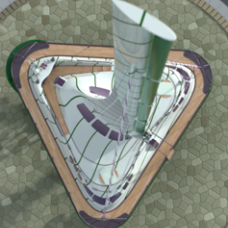# Exponential map algorithm gives bug in case of sphere, need solution to fix issue

## Recommended PostsI am trying to decal sketch on mesh using exponential map. While generating exponential map, I am calculating plane by initial point on mesh and normal calculated on mesh by that point. then algorithm calculates nearest vertices from that point and traverse to them by edges.

Then Edges are projected on plan with their original lengths. It works in most cases, But there is problems in case of sphere. the map is getting twisted.

Do I need to do anything different for the spheres? Any ideas on how this issue can be resolved? Or has anybody done something similar using a different algorithm for exponential map?

I have created below code

while (i m_iExpPos = i; if (pVertex->m_iNeighbour > -1) { CVector vecPoint = pVertex->toVector() - pVertex->Neighbour()->toVector(); double len = vecPoint.norm(); CVector tmp = (pVertex->Neighbour()->normal() * -1) * vecPoint; vec = tmp * sketch.m_meshNorm; vec.unitize(); vec = vec * len; double u = vec.dot(sketch.m_meshX); pVertex->m_u = u + pVertex->Neighbour()->m_u; double v = vec.dot(yDir); pVertex->m_v = v + pVertex->Neighbour()->m_v; } else { vec = pVertex->toVector() - pnt; pVertex->m_u = vec.dot(sketch.m_meshX); pVertex->m_v = vec.dot(yDir); pVertex->m_Propogation = vec; } AddAdjacentUnvisitedVertices(pVertex, lstVerts); } pnt is one point where exponential map will start. Initially, lstVerts will contain vertices near to pnt. Gradually it will add adjacent vertices.

I think problem is algo uses edge length as it is in exponential map. I think it need to be adjusted. I don't know how?

Edited by Kalpesh30

##### Share on other sitesIs the problem due to Singularities?

## Join the conversation

You can post now and register later. If you have an account, sign in now to post with your account.
Note: Your post will require moderator approval before it will be visible.×   Pasted as rich text.   Paste as plain text instead

Only 75 emoji are allowed.